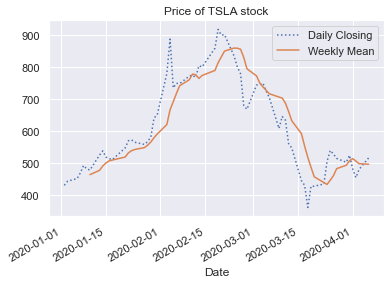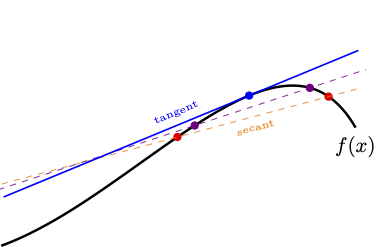# Approximating the derivative¶

April 7, 2020

In calculus, you learnt about the derivative and its central role in modeling processes where a rate of change is important. How do we compute the derivate on a computer?

Recall what you did in your first calculus course to compute the derivative. You memorized derivatives of simple functions like $\cos x, \sin x, \exp x, x^n$ etc. Then you learnt rules like product rule, quotient rule, chain rule etc. In the end you could systematically compute derivatives of complicated functions by reducing it to simpler components and applying the rules. We could teach the computer the same rules and come up with an algorithm for computing derivatives. This is the idea behind automatic differentiation. Python modules like sympy can compute derivatives symbolically in this fashion. However, this approach has its limits.

In the real world, we often encounter complicated functions, such as functions that cannot be represented in terms of simple component functions, or functions whose values you can only query from some proprietary company code, or functions whose values are based off a table, like for instance this function.This function represents Tesla's stock prices this year until yesterday (which I got, in case you are curious, using just a few lines of python code). The function is complicated (not to mention depressing - it reflects the market downturn due to the pandemic). But its rate of change drives some investment decisions. Instead of the oscillatory daily stock values, analysts often look at the rate of change of trend lines (like the rolling weekly means above), a function certainly not expressible in terms of a few simple functions like sines or cosines.

In this activity, we look at computing a numerical approximation to the derivative using something you learnt in calculus.

## Numerical differentiation¶

Suppose $f$ is a function of a single real variable $x$. Its derivative at any point $x$ is the slope of the tangent of its graph at $x$. This slope, as you no doubt recall from calculus, can be numerically approximated by the slope of a secant line:

$$f'(x) \approx {f(x+h/2) - f(x-h/2) \over h}$$

Below is a plot of the tangent line of some function $f$ at $x$, whose slope is $f'(x)$, together with the secant line whose slope is the approximation on the right hand side above. Clearly as the spacing $h$ decreases, the secant line becomes a better and better approximation to the tangent line.The right hand side formula $${f(x+h/2) - f(x-h/2) \over h}$$ can be implemented in python as long as we can compute the values $f(x+h/2)$ and $f(x-h/2)$. As $h \to 0$, we should a good obtain approximation to $f'(x)$.

## Second derivative¶

We take one further step and approximate the second derivative by \begin{aligned} f''(x) & \approx { f'(x+h/2) - f'(x-h/2) \over h } \\ & \approx { \left(\frac{f(x+h/2+h/2) - f(x+h/2-h/2)}{h}\right) - \left(\frac{f(x-h/2) - f(x-h/2-h/2)}{h} \right)\over h } \\ & \approx {f(x+h) - 2f(x) + f(x-h)\over h^2} \end{aligned} This is the Central Difference Formula for the second derivative.

The first task in this activity is to write a function to compute the above-stated second derivative approximation, $${f(x-h) - 2f(x) + f(x+h)\over h^2}$$ given any function $f$ of a single variable $x$. The parameter $h$ should also be input, but can take a default value of $10^{-6}$.

The prerequisite reading for this activity included python functions, keyword arguments, positional arguments, and lambda functions. Let's apply all of these concepts while computing the derivative approximation. Note that python allows you to pass functions themselves as arguments to other functions. Therefore, without knowing what specific function $f$ to apply the central difference formula, we can write a generic function D2 for implementing the formula for any $f$.

In :
def D2(f, x, h=1E-6):
return (f(x-h) - 2*f(x) + f(x+h)) / (h*h)


Let's apply the formula to some nice function, say the sine function.

In :
from math import sin

D2(sin, 0.2)

Out:
-0.19864665468105613

Of course we know that second derivative of $\sin(x)$ is negative of itself, so a quick test of correctness is to compare the above value to that of $-\sin(0.2)$.

In :
-sin(0.2)

Out:
-0.19866933079506122

How do we apply D2 to, say, $\sin(2x)$? One way is to define a function returning $\sin(2*x)$ and then pass it to D2, as follows.

In :
def g(x):
return sin(2*x)

D2(g, 0.2)

Out:
-1.5576429035490946

An alternate way is using a lambda function. This gives a one-liner without damaging code readability.

In :
D2(lambda x: sin(2*x), 0.2)  # central diff approximation

Out:
-1.5576429035490946

Of course, in either case the computed value approximates the actual value of $\sin''(2x) = -4 \sin(2x)$, thus verifying our code.

In :
-4*sin(2* 0.2)               # actual 2nd derivative value

Out:
-1.557673369234602

## Error¶

The error in the approximation formula we just implemented is $$\varepsilon(x) = f''(x) - {f(x-h) - 2f(x) + f(x+h)\over h^2}$$ Although we can't know the error $\varepsilon(x)$ without knowing the true value $f''(x)$, calculus gives you all the tools to bound this error.

Substituting the Taylor expansions $$f(x+h) = f(x) + h f'(x) + \frac{h^2}{2} f''(x) + \frac{h^3}{6} f'''(x) + \frac{h^4}{24} f''''(x) + \cdots$$ and $$f(x-h) = f(x) - h f'(x) + \frac{h^2}{2} f''(x) - \frac{h^3}{6} f'''(x) + \frac{h^4}{24} f''''(x) + \cdots$$ into the definition of $\varepsilon(x)$, we find that the after several cancellations, the dominant term is $O(h^2)$ as $h\to 0$.

This means that if $h$ is halved, the error should decrease by a factor of $4$. Let us take a look at the error in the derivative approximations applied to a simple function $$f(x) = x^{-6}$$ at, say $x=1$. I am sure you can compute the exact derivative using your calculus knowledge. In the code below, we subtract this exact derivative from the computed derivative approximation to obtain the error.

In :
print(' h     D2 Result  Error')
for k in range(4,8):
h = 2**(-k)
d2g = D2(lambda x: x**-6, 1, h=h)
e = d2g - 42
print('%.0e  %.5f  %7.6f' %(h, d2g, e))

 h     D2 Result  Error
6e-02  42.99863  0.998629
3e-02  42.24698  0.246977
2e-02  42.06158  0.061579
8e-03  42.01538  0.015384


Clearly, we observe that the error decreases by a factor of 4 when $h$ is halved. This is in accordance with what we expected from the Taylor expansion analysis above.

## Limitations¶

A serious limitation of numerical differentiation formulas like this can be seen when we take values of $h$ really close to 0. Although the limiting process in calculus relies on $h$ going to 0, your computer is not equipped to deal with very small numbers. This creates issues. Instead of halving $h$, let us aggressively reduce $h$ by a factor of 10, going down to $10^{-13}$ and look at the results.

In :
for k in range(1,14):
h = 10**(-k)
d2g = D2(lambda x: x**-6,1, h)
print('%.0e %18.5f' %(h, d2g))

1e-01           44.61504
1e-02           42.02521
1e-03           42.00025
1e-04           42.00000
1e-05           41.99999
1e-06           42.00074
1e-07           41.94423
1e-08           47.73959
1e-09         -666.13381
1e-10            0.00000
1e-11            0.00000
1e-12   -666133814.77509
1e-13  66613381477.50939


Although a mathematical argument led us to expect better approximations as $h\to 0$, we find that the results from our computer for $h < 10^{-8}$ are totally wrong! The problem is that computers cannot do exact arithmetic: the infinite real number system is replaced by a finite set of numbers allowed in the so-called IEEE standard. This causes errors, called round-off errors that are different from the approximation error $\varepsilon(x)$ we discussed. Specifically, what happened was that for small $h$ we subtracted very closeby numbers, creating round-off errors; we then multiplied by a big number ($1/h^2$) amplifying these round-off errors. We shall not deal in depth with round-off errors in this course, but it pays to be wary of them.